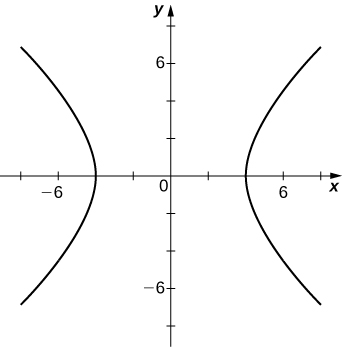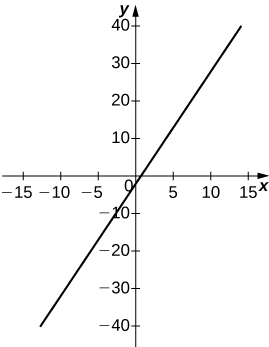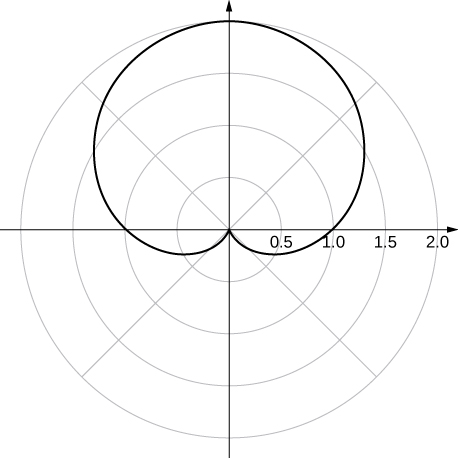$$\newcommand{\id}{\mathrm{id}}$$ $$\newcommand{\Span}{\mathrm{span}}$$ $$\newcommand{\kernel}{\mathrm{null}\,}$$ $$\newcommand{\range}{\mathrm{range}\,}$$ $$\newcommand{\RealPart}{\mathrm{Re}}$$ $$\newcommand{\ImaginaryPart}{\mathrm{Im}}$$ $$\newcommand{\Argument}{\mathrm{Arg}}$$ $$\newcommand{\norm}{\| #1 \|}$$ $$\newcommand{\inner}{\langle #1, #2 \rangle}$$ $$\newcommand{\Span}{\mathrm{span}}$$

# 4.3E: Excersises

$$\newcommand{\vecs}{\overset { \rightharpoonup} {\mathbf{#1}} }$$ $$\newcommand{\vecd}{\overset{-\!-\!\rightharpoonup}{\vphantom{a}\smash {#1}}}$$$$\newcommand{\id}{\mathrm{id}}$$ $$\newcommand{\Span}{\mathrm{span}}$$ $$\newcommand{\kernel}{\mathrm{null}\,}$$ $$\newcommand{\range}{\mathrm{range}\,}$$ $$\newcommand{\RealPart}{\mathrm{Re}}$$ $$\newcommand{\ImaginaryPart}{\mathrm{Im}}$$ $$\newcommand{\Argument}{\mathrm{Arg}}$$ $$\newcommand{\norm}{\| #1 \|}$$ $$\newcommand{\inner}{\langle #1, #2 \rangle}$$ $$\newcommand{\Span}{\mathrm{span}}$$ $$\newcommand{\id}{\mathrm{id}}$$ $$\newcommand{\Span}{\mathrm{span}}$$ $$\newcommand{\kernel}{\mathrm{null}\,}$$ $$\newcommand{\range}{\mathrm{range}\,}$$ $$\newcommand{\RealPart}{\mathrm{Re}}$$ $$\newcommand{\ImaginaryPart}{\mathrm{Im}}$$ $$\newcommand{\Argument}{\mathrm{Arg}}$$ $$\newcommand{\norm}{\| #1 \|}$$ $$\newcommand{\inner}{\langle #1, #2 \rangle}$$ $$\newcommand{\Span}{\mathrm{span}}$$

## Polar Coordinates

Exercise $$\PageIndex{1}$$

In the following exercises, plot the point whose polar coordinates are given by first constructing the angle $$\displaystyle θ$$ and then marking off the distance r along the ray.

1) $$\displaystyle (3,\frac{π}{6})$$

2) $$\displaystyle (−2,\frac{5π}{3})$$

3) $$\displaystyle (0,\frac{7π}{6})$$

4) $$\displaystyle (−4,\frac{3π}{4})$$

5) $$\displaystyle (1,\frac{π}{4})$$

6) $$\displaystyle (2,\frac{5π}{6})$$

7) $$\displaystyle (1,\frac{π}{2})$$

Solution 1:Solution 3:Solution 5:Solution 7:Exercise $$\PageIndex{2}$$

For the following exercises, consider the polar graph below. Give two sets of polar coordinates for each point.8) Coordinates of point A.

9) Coordinates of point B.

10) Coordinates of point C.

11) Coordinates of point D.

Solution 9: $$\displaystyle B(3,\frac{−π}{3}) B(−3,\frac{2π}{3})$$,

Solution 11: $$\displaystyle D(5,\frac{7π}{6}) D(−5,\frac{π}{6})$$

Exercise $$\PageIndex{3}$$

For the following exercises, the rectangular coordinates of a point are given. Find two sets of polar coordinates for the point in $$\displaystyle (0,2π]$$. Round to three decimal places.

12) $$\displaystyle (2,2)$$

13) $$\displaystyle (3,−4) (3, −4)$$

14) $$\displaystyle (8,15)$$

15) $$\displaystyle (−6,8)$$

16) $$\displaystyle (4,3)$$

17) $$\displaystyle (3,−\sqrt{3})$$

Solution 13: $$\displaystyle (5,−0.927)(−5,−0.927+π)$$,

Solution 15: $$\displaystyle (10,−0.927)(−10,−0.927+π)$$,

Solution 17: $$\displaystyle 2\sqrt{3},−0.524)(−2\sqrt{3},−0.524+π)$$

Exercise $$\PageIndex{4}$$

For the following exercises, find rectangular coordinates for the given point in polar coordinates.

18) $$\displaystyle (2,\frac{5π}{4})$$

19) $$\displaystyle (−2,\frac{π}{6})$$

20) $$\displaystyle (5,\frac{π}{3})$$

21) $$\displaystyle (1,\frac{7π}{6})$$

22) $$\displaystyle (−3,\frac{3π}{4})$$

23) $$\displaystyle (0,\frac{π}{2})$$

24) $$\displaystyle (−4.5,6.5)$$

Solution 19: $$\displaystyle −\sqrt{3},−1)$$,

Solution 21: $$\displaystyle (−\frac{\sqrt{3}}{2},\frac{−1}{2})$$,

Solution 23: $$\displaystyle (0,0)$$

Exercise $$\PageIndex{5}$$

For the following exercises, determine whether the graphs of the polar equation are symmetric with respect to the $$\displaystyle x$$-axis, the $$\displaystyle y$$ -axis, or the origin.

25) $$\displaystyle r=3sin(2θ)$$

26) $$\displaystyle r^2=9cosθ$$

27) $$\displaystyle r=cos(\frac{θ}{5})$$

28) $$\displaystyle r=2secθ$$

29) $$\displaystyle r=1+cosθ$$

Solution 25: Symmetry with respect to the x-axis, y-axis, and origin,

Solution 27: Symmetric with respect to x-axis only,

Solution 29: Symmetry with respect to x-axis only.

Exercise $$\PageIndex{6}$$

For the following exercises, describe the graph of each polar equation. Confirm each description by converting into a rectangular equation.

30) $$\displaystyle r=3$$

31) $$\displaystyle θ=\frac{π}{4}$$

32) $$\displaystyle r=secθ$$

33) $$\displaystyle r=cscθ$$

Solution 31: Line $$\displaystyle y=x$$,

Solution 33: $$\displaystyle y=1$$

Exercise $$\PageIndex{7}$$

For the following exercises, convert the rectangular equation to polar form and sketch its graph.

34) $$\displaystyle x^2+y^2=16$$

35) $$\displaystyle x^2−y^2=16$$

36) $$\displaystyle x=8$$

Solution 35: Hyperbola; polar form $$\displaystyle r^2cos(2θ)=16$$ or $$\displaystyle r^2=16secθ.$$Exercise $$\PageIndex{8}$$

For the following exercises, convert the rectangular equation to polar form and sketch its graph.

37) $$\displaystyle 3x−y=2$$

38) $$\displaystyle y^2=4x$$

Solution 37: $$\displaystyle r=\frac{2}{3cosθ−sinθ}$$Exercise $$\PageIndex{9}$$

For the following exercises, convert the polar equation to rectangular form and sketch its graph.

39) $$\displaystyle r=4sinθ$$

40) $$\displaystyle x^2+y^2=4y$$

41) $$\displaystyle r=6cosθ$$

42) $$\displaystyle r=θ$$

43) $$\displaystyle r=cotθcscθ$$

Solution 39:Solution 41: $$\displaystyle xtan\sqrt{x^2+y^2}=y$$Exercise $$\PageIndex{10}$$

For the following exercises, sketch a graph of the polar equation and identify any symmetry.

44) $$\displaystyle r=1+sinθ$$

45) $$\displaystyle r=3−2cosθ$$

46) $$\displaystyle r=2−2sinθ$$

47) $$\displaystyle r=5−4sinθ$$

48) $$\displaystyle r=3cos(2θ)$$

49) $$\displaystyle r=3sin(2θ)$$

50) $$\displaystyle r=2cos(3θ)$$

51) $$\displaystyle r=3cos(\frac{θ}{2})$$

52) $$\displaystyle r^2=4cos(\frac{2}{θ})$$

53) $$\displaystyle r^2=4sinθ$$

54) $$\displaystyle r=2θ$$

Solution 44: y-axis symmetrySolution 46: y-axis symmetrySolution 48: x- and y-axis symmetry and symmetry about the poleSolution 50: x-axis symmetrySolution 52: x- and y-axis symmetry and symmetry about the poleSolution 54: no symmetryExercise $$\PageIndex{11}$$

55) [T] The graph of $$\displaystyle r=2cos(2θ)sec(θ).$$ is called a strophoid. Use a graphing utility to sketch the graph, and, from the graph, determine the asymptote.

56) [T] Use a graphing utility and sketch the graph of $$\displaystyle r=\frac{6}{2sinθ−3cosθ}$$.

57) [T] Use a graphing utility to graph $$\displaystyle r=\frac{1}{1−cosθ}$$.

58) [T] Use technology to graph $$\displaystyle r=e^{sin(θ)}−2cos(4θ)$$.

59) [T] Use technology to plot $$\displaystyle r=sin(\frac{3θ}{7})$$ (use the interval $$\displaystyle 0≤θ≤14π$$).

60) Without using technology, sketch the polar curve $$\displaystyle θ=\frac{2π}{3}$$.

61) [T] Use a graphing utility to plot $$\displaystyle r=θsinθ$$ for $$\displaystyle −π≤θ≤π$$.

62) [T] Use technology to plot $$\displaystyle r=e^{−0.1θ}$$ for $$\displaystyle −10≤θ≤10.$$

63) [T] There is a curve known as the “Black Hole.” Use technology to plot $$\displaystyle r=e^{−0.01θ}$$ for $$\displaystyle −100≤θ≤100$$.

64) [T] Use the results of the preceding two problems to explore the graphs of $$\displaystyle r=e^{−0.001θ}$$ and $$\displaystyle r=e^{−0.0001θ}$$ for $$\displaystyle |θ|>100$$.

Solution 56: a lineSolution 58:Solution 60:Solution 62:Solution 64: Answers vary. One possibility is the spiral lines become closer together and the total number of spirals increases.